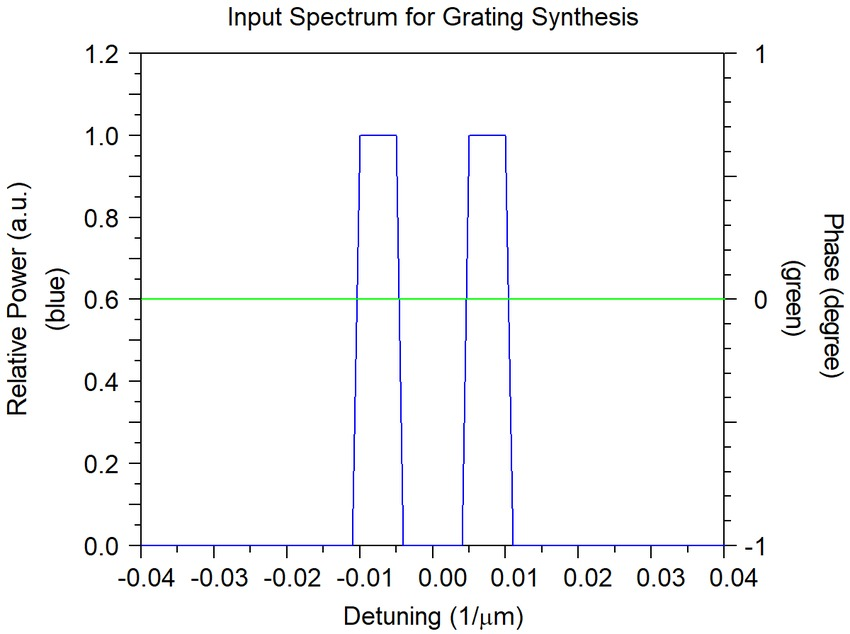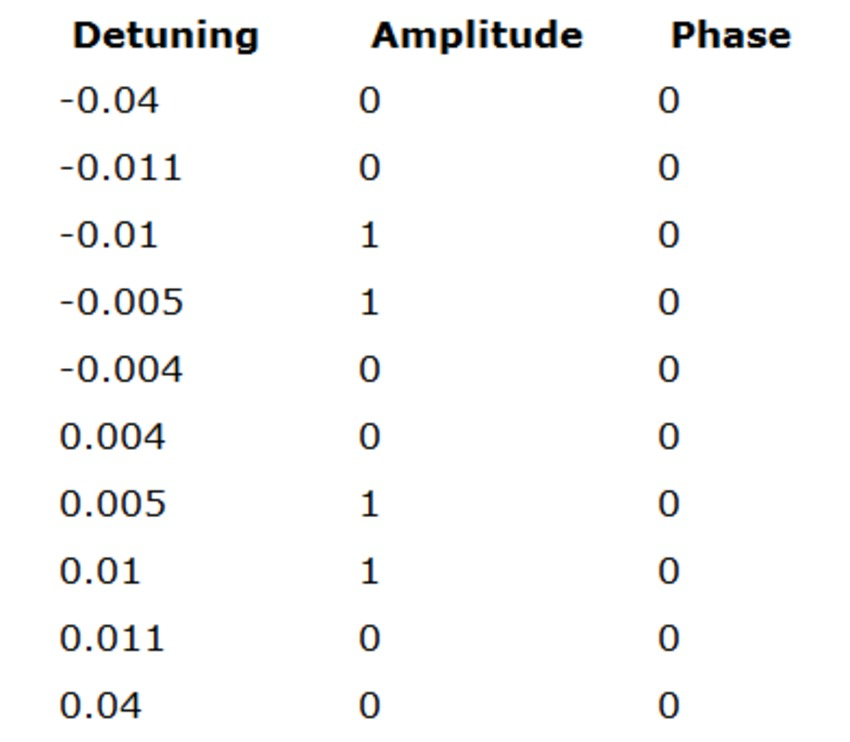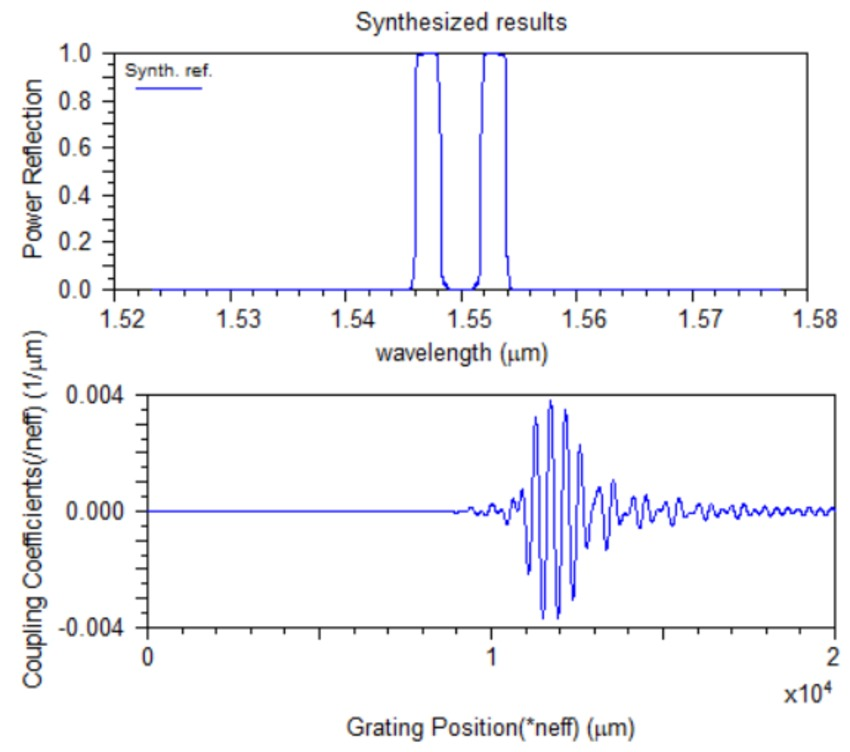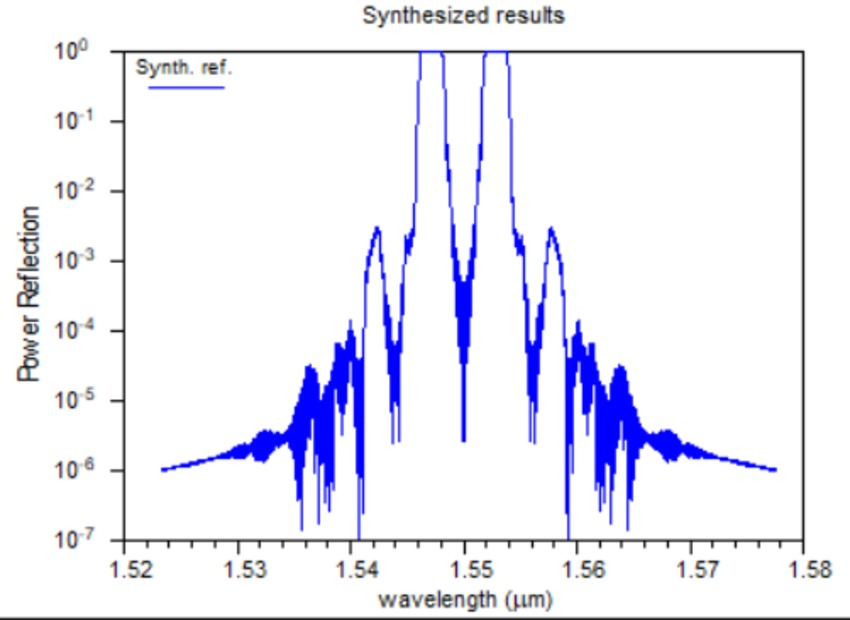# Two Channel Filter

## Introduction

A two-channel filter is useful for detuning. In this application note, we will review the process of synthesizing such a filter in a grating using GratingMOD.

In GratingMOD grating synthesis feature, the response for the system is first defined by the user. Next, GratingMOD will compute the grating characteristics, including index distribution and chirp, for the grating itself.

## Creating The Structure

This filter will be designed to have two channels, centered at a wavelength of 1.55 µm with a bandwidth of about 0.02 µm. The desired spectral performance is shown in Figure 1.Figure 1. Desired spectral performance for two channel filter. Note that the wavelength is defined in reference to the central wavelength, 1.55 µm.

For this design, we will define the two channel spectrum for detuning using a data file representing the data given in Figure 1.Figure 2. Datafile for data contained in Figure 1.

## Performing The Synthesis

Using the above data, the synthesized results as obtained from GratingMOD are shown in Figure 3.Figure 3. Results of the Two Channel Filter simulation (left); The same results shown on a log plot (right).